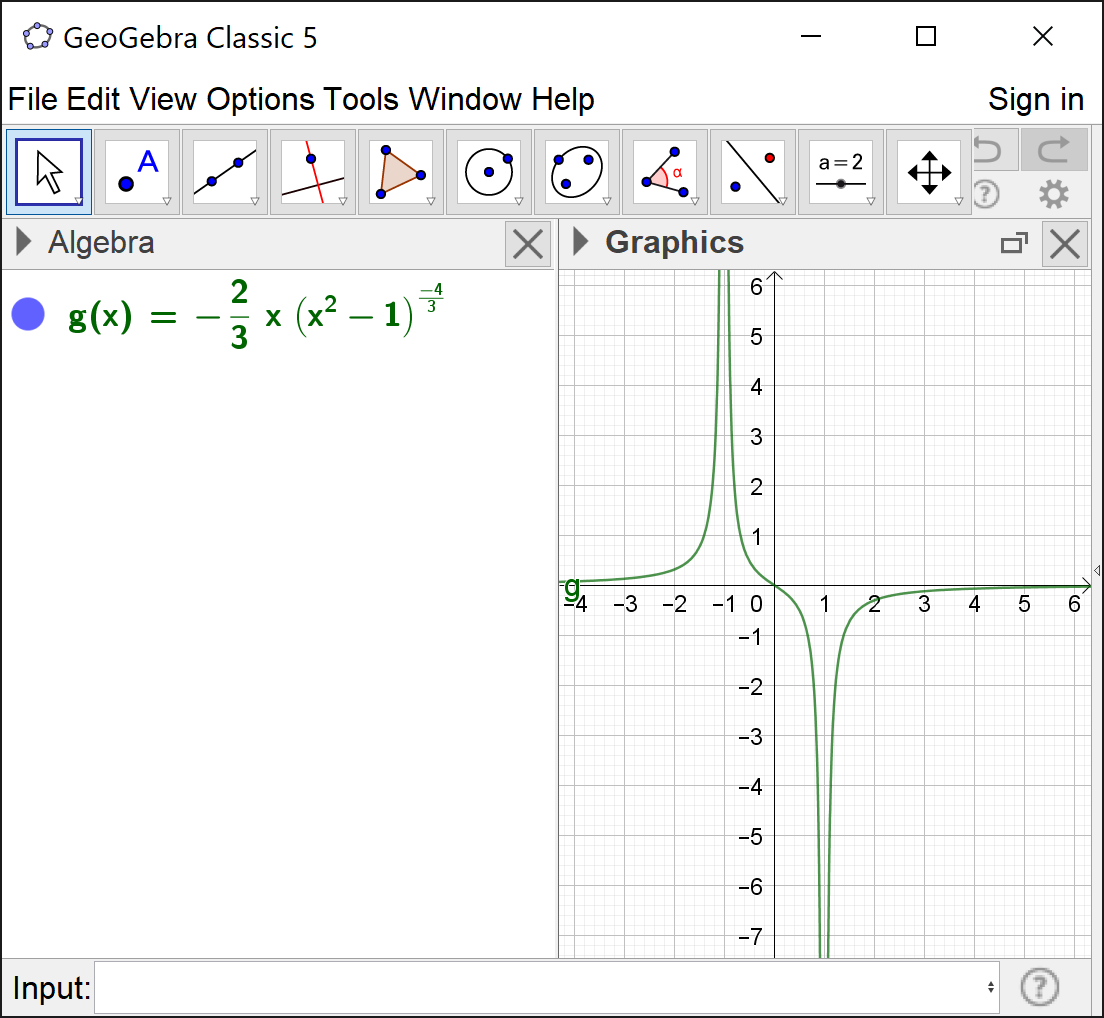# I found a bug

bob mcfakename shared this question 1 year ago

I found a bug with the graphing

g(x)=-((2)/(3)) x (x^(2)-1)^(-((4)/(3)))

and

g(x)=-((2)/(3)) x (x^(2)-1)^((-4)/(3))1

Looks OK to me, which version are you using? (sorry, can't access your screenshot)